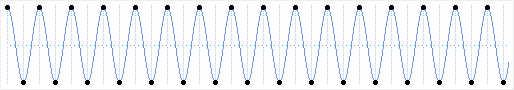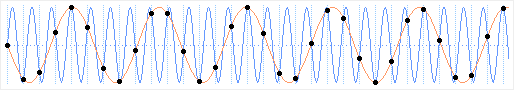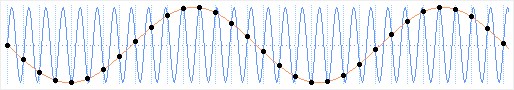# 3. The Nyquist Limit

Home | Next

The simplest sound wave is an oscillation between two amplitudes. A sampled waveform thus needs at least two sample points per cycle. Put another way, the wave's frequency must not be above half the sampling frequency. This limit is called the Nyquist limit of a given sampling frequency.Sine wave at 1/2 sampling rate with two samples per cycle

If a sine wave higher than the Nyquist frequency is sampled, a sine wave of lower frequency results. This effect is called aliasing.Sine waves above 1/2 sampling rate (blue) and resulting aliases (orange)

Since an alias is no different than a normally sampled wave of the same frequency, aliasing can be avoided only by removing frequencies above the Nyquist limit before sampling.